#### File Exchange > Data Analysis >    Compare Datasets and Fit ParametersAuthor:
OriginLab Technical Support
3/25/2016
Last Update:
4/25/2023
282
Total Ratings:
3
File Size:
72 KB
Average Rating: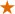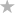File Name:
Compare Da...rs.opx
File Version:
1.70
Minimum Versions:
Free
Summary:

Compare parameters across multiple datasets, or compare multiple datasets using a nonlinear model.

Description:

Purpose
This tool can be used to:

•  Compare parameters across multiple datasets.
• Compare multiple datasets using a nonlinear model.

Two algorithms are provided for comparison: Akaike Information Criteria(AIC) and F-test.

Installation
Download the file Compare Datasets.opx. Then drag-and-drop the file onto the Origin workspace. An app icon will appear in the Apps gallery.

Operation

• Select multiple data columns in a worksheet, or start with a graph of the desired datasets. Then press the App icon to open the dialog
• Select fitting function
• Select whether to compare the datasets, or compare the fit parameters
• Press "Fit Control" button to open a dialog where you further control the fit if required. In this dialog, you can fix parameters, or share parameters between datasets when comparing parameters.  A preview graph with current fit results will also be displayed

Algorithm
When comparing one specific parameter (or datasets), we are actually comparing two models. For one model, the parameter value can vary among different datasets, this is the more complicated model. For the other, the parameter values are assumed to be same for all datasets, this is the simpler model. When comparing a parameter, the more complicated model corresponds to independent fit for each dataset and the simpler model corresponds to global fit with the parameter shared.
When comparing datasets using a fit model, the more complicated model corresponds to independent fit for each dataset while the simpler model corresponds to a function with all parameters shared in all datasets.
1. Akaike Information Criteria(AIC)
For each model, Origin calculates the AIC value by: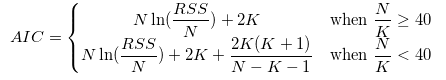where RSS is residual sum of squares for that model, N is the number of data points, K is the number of parameters.
For two fitting models, the one with the smaller AIC value is suggested to be a better model for the datasets, and then we can determine whether parameter values to be compared are same.
We can also make decisions based on the Akaike's weight value, which can be computed as: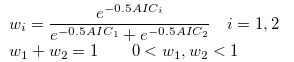Here i=1 represents the simpler model and i=2 represents the more complicated model.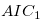and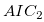are the AIC values of the two fitting models, respectively.
If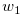is larger than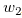, we can conclude that parameter values to be compared are same, otherwise parameter values are different.

2. F-test
Suppose the sum of RSS and the sum of df (degrees of freedom) of the simpler model fit are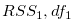and those of the more complicated model fit are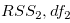.
We can compute the F value by: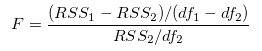Once the F value is computed, Origin calculates the P-value by: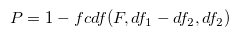This P-value can be used to determine whether parameter values to be compared are different. If the P-value is greater than 0.05, we can conclude that parameter values to be compared are not significantly different.

 12/07/2021 zs036 ,12/07/2021 alialjabe1994 OK06/20/2016 erichdz This App is great; can the datasets be compared without the use of a function? Thank you,